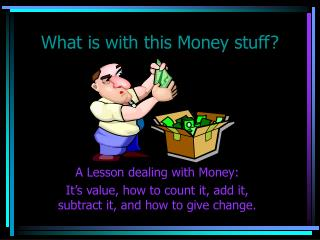DownloadDownload PresentationWhat is with this Money stuff?

# What is with this Money stuff?

Download Presentation## What is with this Money stuff?

- - - - - - - - - - - - - - - - - - - - - - - - - - - E N D - - - - - - - - - - - - - - - - - - - - - - - - - - -
##### Presentation Transcript

1. What is with this Money stuff? A Lesson dealing with Money: It’s value, how to count it, add it, subtract it, and how to give change.

2. What exactly is Money? Money is basically, a medium of exchange. Without it, there would be no easy way to exchange payment for either goods or services. If goods were used for exchange instead of money, there would be many problems.The value to one person of a specific item would be different from another person who doesn’t have as great a need for the item. Using money helps people buy things without the hassle of exchanging goods.

3. Dollar Values The value of a dollar is defined by how many dollars and cents it is worth. The more dollars or cents a dollar is worth, the more valuable it is. The number next to each dollar shows the number of dollars (\$) and cents (¢) it takes to make one of these dollar. To see the dollar values just click on the site below, the value of dollars are pretty self explanatory. Click here to look at some dollar values.

4. Adding Dollars When adding Dollars, just look at the value and count by that amount. Usually it is easiest to start with the highest dollar amount and to work your way down. For Example: How much total do you have if you have 2 Fifty Dollar Bills, 3 Twenty Dollar Bills, 1 Ten Dollar Bills, 3 Five Dollar Bills and 1 One Dollar Bill? • Fifty: 50, 100 • Twenty: 120, 140, 160 • Ten: 170 • Five: 175, 180, 185 • One: 186 Total: \$186

5. Adding Dollars with Coins When dealing with dollars (1 Dollar= 100 cents), it might be easier if you count by hundreds the number of dollars you have. Then starting with this total, you count on with the change you have until you get your total, then you can convert back to dollars. Example: How many cents are 7 dollars, 1 Quarter, 4 Dimes, 3 Nickels and 1 Penny worth? • Count Dollars: 100, 200, 300, 400, 500, 600, 700 • Count Quarter starting with total: 725 • Count Nickels: 730, 735, 740 • Count Dimes: 750, 760, 770, 780 • Count Pennies: 781 Total: 781 cents or \$7.81

6. Adding Money Amounts Amounts of money may be written in several different ways. Cents may be written with the cent sign (¢) and dollars can be written with the dollar sign (\$). Adding money that is expressed in these forms just involves adding the amounts and placing the proper sign on the answer. Often money is written as a decimal with dollars to the left of the decimal point and cents to the right of the decimal point (as shown in earlier examples). For example: Twenty-three dollars and eighty-seven cents is written out as \$23.87.

7. Decimal money amounts are added the same way the decimals are added. Remember to put the \$ sign before the answer. Adding Decimals is just like adding other numbers. Always line up the decimal when adding decimals. Remember to put the decimal point in the proper place in your answer. Here are some examples: \$1.25 + .83 ------- \$2.08 \$14.75 + 2.23 --------- \$16.98 \$22.18 + 8.50 --------- \$30.68 \$19.25 +13.99 --------- \$33.24

8. Subtracting Money Subtracting money that is expressed in the forms of cent and dollar signs just involves the amounts and placing the proper sign on the answer. It goes the same way as adding money, except you subtract. Just make sure you line up the decimals before subtracting and to remember when you’re done with the problem that you put the decimal in the correct spot and that you use a \$ sign. Here are some examples: \$1.25 - .83 ------- \$ 0.42 \$14.75 - 2.23 --------- \$12.52 \$22.18 - 8.50 --------- \$13.68 \$19.25 -13.99 --------- \$ 5.26

9. Giving Change Change is the money a customer receives back when they have made a purchase. Often the customer gives the merchant more money than the amount due because they may not have the exact change or don’t want to bother with finding it. Therefore, the merchant needs to determine how much extra was paid and return the excess, which is called change.

10. When the merchant gives change back to the customer, he or she wants to give the least number of coins in change. How to find the Least Number of Coins to give in Change: • Determine the total amount of change due. • Start with the highest denomination of coins or bills. • Use as many of this coin as possible without exceeding the amount of change due. • Repeat this process with the next lowest denomination of coin or bill. Click here to see an example.

11. The process of giving money back accomplishes the following: • The customer has the least possible coins in their purse or pocket • The amount of money returned is double checked, when it is gathered and when given to the customer • The amount of change due is double checked by counting change • The possibility of a misunderstanding is eliminated • The merchant and the customer are both treated fairly by making sure the change is exact Click here to see an example of counting back.

12. Where To Go Now? Back to First Slide of Money Presentation Lesson Page Back to Money Lesson Main Page